# Drawing a Perpendicular to a Line at a Point on the Line

#### description

• Method using ruler and compasses
• Method using a ruler and a set-square
• Method using a ruler and a protractor

# Perpendicular to a line through a point on it:

• Given a line l drawn on a paper sheet and a point P lying on the line. It is easy to have perpendicular to l through P. We can simply fold the paper such that the lines on both sides of the fold overlap each other.
• Tracing paper or any transparent paper could be better for this activity. Let us take such a paper and draw any line l on it. Let us mark a point P anywhere on l.
• Fold the sheet such that l is reflected on itself; adjust the fold so that the crease passes through the marked point P. Open out; the crease is perpendicular to l.

## A. Method using ruler and compasses:

As is the preferred practice in Geometry, the dropping of a perpendicular can be achieved through the “ruler-compasses” construction as follows :

Step 1: Given a point P on a line l.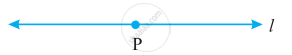Step 2: With P as a centre and a convenient radius, construct an arc intersecting the line l at two points A and B.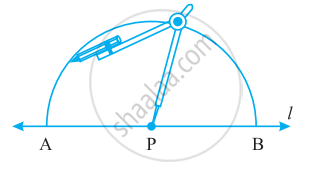Step 3: With A and B as centers and a radius greater than APconstruct two arcs, which cut each other at Q.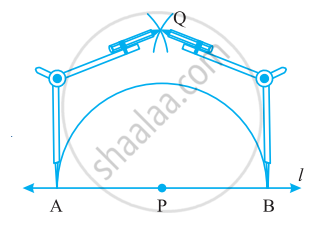Step 4: Join PQ. Then bar"PQ" is perpendicular to l.

We write bar"PQ" ⊥ l.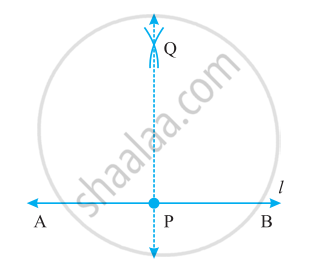## B. Method using a ruler and a set-square:

Step 1: A line l and a point P are given. Note that P is on line l.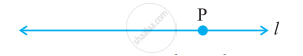Step 2: Place a ruler with one of its edges along l. Hold this firmly.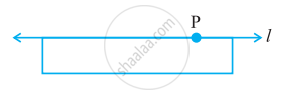Step 3: Place a set-square with one of its edges along the already aligned edge of the ruler such that the right-angled corner is in contact with the ruler.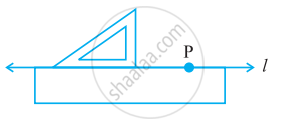Step 4: Slide the set-square along the edge of the ruler until its right-angled corner coincides with P.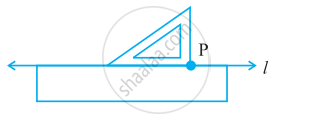Step 5: Hold the set-square firmly in this position. Draw bar"PQ" along the edge of the set-square.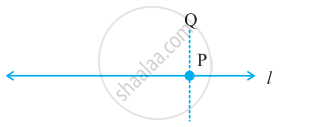bar"PQ" is perpendicular to l.

## C. Method using a ruler and a protractor:

• Draw line RS. Take point M anywhere on the line.
• In order to draw a perpendicular through M, place the centre of the protractor on point M, as shown.
•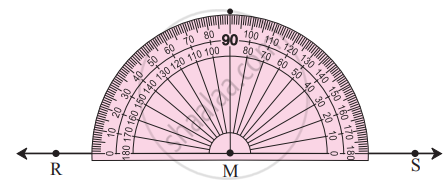• Mark a point N at the 90° mark on the protractor.
• Draw a line passing through points M and N.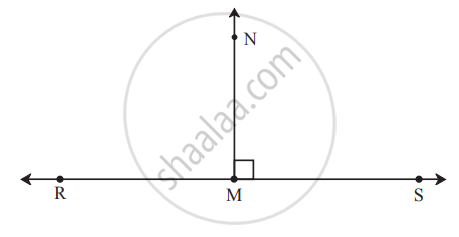• The line MN is perpendicular to line RS at M.
Line MN ⊥ line RS.
If you would like to contribute notes or other learning material, please submit them using the button below.

### Shaalaa.com

Perpendicular to a Line Through Point on the Line [00:02:52]
S
0%